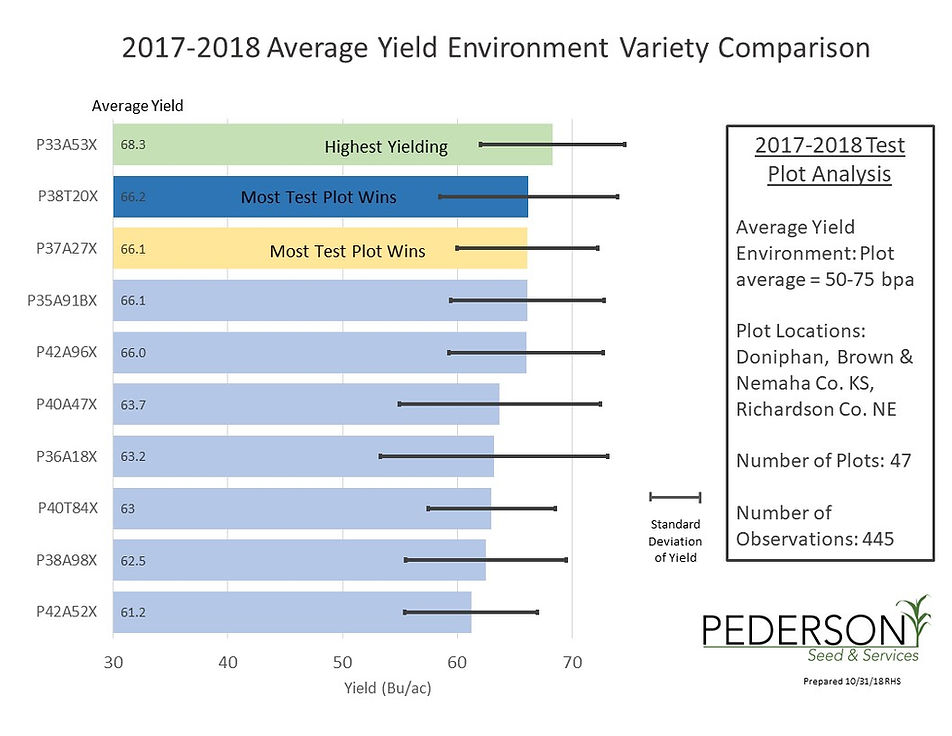top of page
2018 Corn Hybrid Test Plot Analysi
###### 2018 Test Plot Analysis: Explained

Below are test plot results from 2017 and 2018 for corn and soybean test plots from Brown, Nemaha, Doniphan, and Richardson Counties.

Graphs are grouped into three yield zones, high, average, and low. In each grouping, a highest yielding, a most consistent yielding, and a most test plot wins designation has been highlighted. Hybrids with less than 3 observations were not included in data calculations. Plot location, number of plots, and number of individual observations is located on the right side of each graph. One set of graphs shows the percent wins by each hybrid in each yield category. This is showing what percentage of the time a hybrid won in that yield environment looking only at Pioneer products in each test plot.

Finally, a standard deviation bar is displayed on each hybrid bar, depicting the extent of deviation from the hybrid average. Essentially this bar tells us how close together, or far apart from the average the data points were grouped. Smaller standard deviation values suggest the hybrid average was more consistent, with the majority of data points all very similar. Higher standard deviations suggest that there is more variability in the results for the hybrid and that there were more extreme results for that hybrid. If you look at one of the graphs you can turn this bar into a bushel per acre range that the hybrid normally fell in. For the first hybrid in the first graph, yield values for that hybrid typically fell between 225-275 bushels per acre.

## 2018 Corn Hybrid Test Plot Analysis2018 Soybean Variet Test Plot Analysis

## 2018 Soybean Variety Test Plot Analysisbottom of page